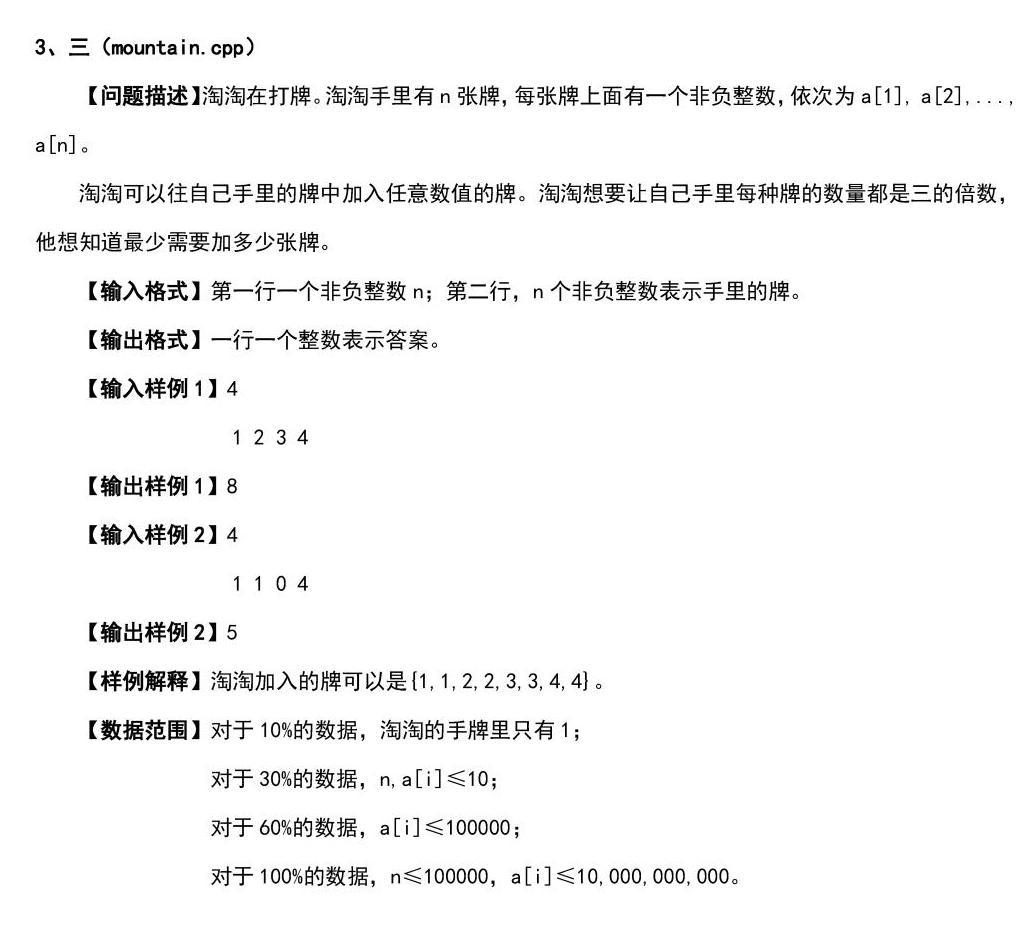#### 菜鸟程序员等　　级 排　　名 4135 经　　验 1295 参赛次数 4 文章发表 15 年　　龄 12 在职情况 学生 学　　校 飞龙实验小学 专　　业 数学课代表

自我简介：

#### TA的其他文章

 [编程入门]自定义函数之字符串反转浏览：16 亲和数（判约数的基本功）浏览：14 C语言训练-计算一个整数N的阶乘（可用函数，也可以不用）浏览：14

#### 你可能喜欢for(int i=0;i<n;i++)
{
cin>>a;
b[a]++;
}

for(int i=0;i<=n;i++)
{
for(int j=0;j<=n;j++)
{
if(b[i]%3!=0)
{
b[i]++;
s++;
}
}
}

#include<bits/stdc++.h>
using namespace std;
int a,n,b,s=0;;
void pan()
{
cin>>n;
for(int i=0;i<n;i++)
{
cin>>a;
b[a]++;
}
for(int i=0;i<=n;i++)
{
for(int j=0;j<=n;j++)
{
if(b[i]%3!=0)
{
b[i]++;
s++;
}
}
}
cout<<s;
}
int main()
{
pan();

return 0;
}

0.0分

1 人评分